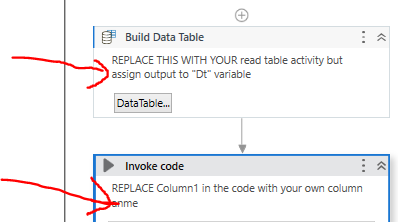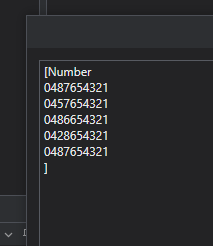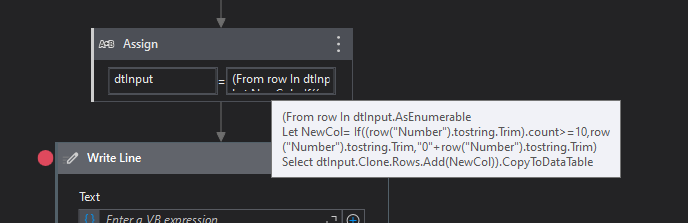# Add 0 in the beginning of a mobile number if the mobile number only has 9 digits and starts with 4

Hi,

I want add a 0 in the beginning for all the mobile numbers in a phone number column of a data table if the mobile number only has 9 digits and starts with 4. I’m basically cleaning up the phone number column.
For instance if the mobile number is 0412345678, then no action required.
If the mobile number is 4312345678, then the result should be 0434182062
As I am having around 70,000 rows, I don’t want to use a for each. Please help if anyone has a solution for this.

refer to this sequence @ammohan

uipathF.xaml (11.1 KB)

and take note of the annotationsRefere this sequence and take input variable type string.
If it works mark as solution
In if condition Use this Input.Length=9 And Input.StartsWith(“4”)
For Excel refer this xaml file
ReplaceMobileNo.zip (9.1 KB)

Hi @ammohan,

InputOutputWorkflowCode

``````(From row In dtInput.AsEnumerable
Let NewCol= If((row("Number").tostring.Trim).count>=10,row("Number").tostring.Trim,"0"+row("Number").tostring.Trim)